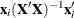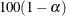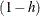The REG Procedure

OUTPUT Statement

• OUTPUT <OUT=SAS-data-set> <keyword=names> <…keyword=names>;

The OUTPUT statement creates a new SAS data set that saves diagnostic measures calculated after fitting the model. The OUTPUT statement refers to the most recent MODEL statement. At least one keyword=names specification is required.

All the variables in the original data set are included in the new data set, along with variables created in the OUTPUT statement. These new variables contain the values of a variety of statistics and diagnostic measures that are calculated for each observation in the data set. If you want to create a SAS data set in a permanent library, you must specify a two-level name. For more information about permanent libraries and SAS data sets, see SAS Language Reference: Concepts.

The OUTPUT statement cannot be used when a TYPE=CORR, TYPE=COV, or TYPE=SSCP data set is used as the input data set for PROC REG. See the section Input Data Sets for more details.

The statistics created in the OUTPUT statement are described in this section. More details are given in the section Predicted and Residual Values and the section Influence Statistics. Also see Chapter 4: Introduction to Regression Procedures, for definitions of the statistics available from the REG procedure.

You can specify the following options in the OUTPUT statement:

OUT=SAS data set

gives the name of the new data set. By default, the procedure uses the DATAn convention to name the new data set.

keyword=names

specifies the statistics to include in the output data set and names the new variables that contain the statistics. Specify a keyword for each desired statistic (see the following list of keywords), an equal sign, and the variable or variables to contain the statistic.

In the output data set, the first variable listed after a keyword in the OUTPUT statement contains that statistic for the first dependent variable listed in the MODEL statement; the second variable contains the statistic for the second dependent variable in the MODEL statement, and so on. The list of variables following the equal sign can be shorter than the list of dependent variables in the MODEL statement. In this case, the procedure creates the new names in order of the dependent variables in the MODEL statement.

For example, the following SAS statements create an output data set named b:

proc reg data=a;
model y z=x1 x2;
output out=b
p=yhat zhat
r=yresid zresid;
run;


In addition to the variables in the input data set, b contains the following variables:

• yhat, with values that are predicted values of the dependent variable y

• zhat, with values that are predicted values of the dependent variable z

• yresid, with values that are the residual values of y

• zresid, with values that are the residual values of z

You can specify the following keywords in the OUTPUT statement. See the section Model Fit and Diagnostic Statistics for computational formulas.

Table 97.6: Keywords for OUTPUT Statement

Keyword

Description

COOKD=names

Cook’s D influence statistic

COVRATIO=names

standard influence of observation on covariance of betas, as
discussed in the section Influence Statistics

DFFITS=names

standard influence of observation on predicted value

H=names

leverage,LCL=names

lower bound of a% confidence interval for an
individual prediction. This includes the variance of the
error, as well as the variance of the parameter estimates.

LCLM=names

lower bound of a% confidence interval for the
expected value (mean) of the dependent variable

PREDICTED | P=names

predicted values

PRESS=names

ith residual divided by, where h is the leverage,
and where the model has been refit without the ith
observation

RESIDUAL | R=names

residuals, calculated as ACTUAL minus PREDICTED

RSTUDENT=names

a studentized residual with the current observation deleted

STDI=names

standard error of the individual predicted value

STDP=names

standard error of the mean predicted value

STDR=names

standard error of the residual

STUDENT=names

studentized residuals, which are the residuals divided by their
standard errors

UCL=names

upper bound of a% confidence interval for an
individual prediction

UCLM=names

upper bound of a% confidence interval for the
expected value (mean) of the dependent variable